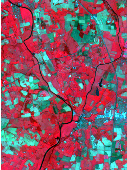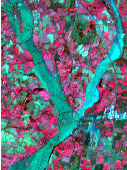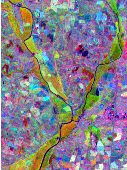## Chapter 21Change Detection

### 21.1 Introduction

Change detection techniques try to detect and locate areas which have changed between two or more observations of the same scene. These changes can be of different types, with different origins and of different temporal length. This allows to distinguish different kinds of applications:

• land use monitoring, which corresponds to the characterization of the evolution of the vegetation, or its seasonal changes;
• natural resources management, which corresponds mainly to the characterization of the evolution of the urban areas, the evolution of the deforestation, etc.
• damage mapping, which corresponds to the location of damages caused by natural or industrial disasters.

From the point of view of the observed phenomena, one can distinguish 2 types of changes whose nature is rather different: the abrupt changes and the progressive changes, which can eventually be periodic. From the data point of view, one can have:

• Image pairs before and after the event. The applications are mainly the abrupt changes.
• Multi-temporal image series on which 2 types on changes may appear:
• The slow changes like for instance the erosion, vegetation evolution, etc. The knowledge of the studied phenomena and of their consequences on the geometrical and radiometrical evolution at the different dates is a very important information for this kind of analysis.
• The abrupt changes may pose different kinds of problems depending on whether the date of the change is known in the image series or not. The detection of areas affected by a change occurred at a known date may exploit this a priori information in order to split the image series into two sub-series (before an after) and use the temporal redundancy in order to improve the detection results. On the other hand, when the date of the change is not known, the problem has a higher difficulty.

From this classification of the different types of problems, one can infer 4 cases for which one can look for algorithms as a function of the available data:

1. Abrupt changes in an image pair. This is no doubt the field for which more work has been done. One can find tools at the 3 classical levels of image processing: data level (differences, ratios, with or without pre-filtering, etc.), feature level (edges, targets, etc.), and interpretation level (post-classification comparison).
2. Abrupt changes within an image series and a known date. One can rely on bi-date techniques, either by fusing the images into 2 stacks (before and after), or by fusing the results obtained by different image couples (one after and one before the event). One can also use specific discontinuity detection techniques to be applied in the temporal axis.
3. Abrupt changes within an image series and an unknown date. This case can be seen either as a generalization of the preceding one (testing the N-1 positions for N dates) or as a particular case of the following one.
4. Progressive changes within an image series. One can work in two steps:
1. detect the change areas using stability criteria in the temporal areas;
2. identify the changes using prior information about the type of changes of interest.

#### 21.1.1 Surface-based approaches

In this section we discuss about the damage assessment techniques which can be applied when only two images (before/after) are available.

As it has been shown in recent review works , a relatively high number of methods exist, but most of them have been developed for optical and infrared sensors. Only a few recent works on change detection with radar images exist . However, the intrinsic limits of passive sensors, mainly related to their dependence on meteorological and illumination conditions, impose severe constraints for operational applications. The principal difficulties related to change detection are of four types:

1. In the case of radar images, the speckle noise makes the image exploitation difficult.
2. The geometric configuration of the image acquisition can produce images which are difficult to compare.
3. Also, the temporal gap between the two acquisitions and thus the sensor aging and the inter-calibration are sources of variability which are difficult to deal with.
4. Finally, the normal evolution of the observed scenes must not be confused with the changes of interest.

The problem of detecting abrupt changes between a pair of images is the following: Let I1,I2 be two images acquired at different dates t1,t2; we aim at producing a thematic map which shows the areas where changes have taken place.

Three main categories of methods exist:

• Strategy 1: Post Classification Comparison

The principle of this approach  is two obtain two land-use maps independently for each date and comparing them.

• Strategy 2: Joint classification

This method consists in producing the change map directly from a joint classification of both images.

• Strategy 3: Simple detectors

The last approach consists in producing an image of change likelihood (by differences, ratios or any other approach) and thresholding it in order to produce the change map.

Because of its simplicity and its low computation overhead, the third strategy is the one which has been chosen for the processing presented here.

### 21.2 Change Detection Framework

The source code for this example can be found in the file
Examples/ChangeDetection/ChangeDetectionFrameworkExample.cxx.

This example illustrates the Change Detector framework implemented in OTB. This framework uses the generic programming approach. All change detection filters are otb::BinaryFunctorNeighborhoodImageFilter s, that is, they are filters taking two images as input and provide one image as output. The change detection computation itself is performed on the neighborhood of each pixel of the input images.

The first step in building a change detection filter is to include the header of the parent class.

#include "otbBinaryFunctorNeighborhoodImageFilter.h"

The change detection operation itself is one of the templates of the change detection filters and takes the form of a function, that is, something accepting the syntax foo(). This can be implemented using classical C/C++ functions, but it is preferable to implement it using C++ functors. These are classical C++ classes which overload the () operator. This allows to be used with the same syntax as C/C++ functions.

Since change detectors operate on neighborhoods, the functor call will take 2 arguments which are itk::ConstNeighborhoodIterator s.

The change detector functor is templated over the types of the input iterators and the output result type. The core of the change detection is implemented in the operator() section.

template<class TInput1, class TInput2, class TOutput>
class MyChangeDetector
{
public:
// The constructor and destructor.
MyChangeDetector() {}
~MyChangeDetector() {}
// Change detection operation
inline TOutput operator ()(const TInput1& itA,
const TInput2& itB)
{

TOutput result = 0.0;

for (unsigned long pos = 0; pos < itA.Size(); ++pos)
{

result += static_cast<TOutput>(itA.GetPixel(pos) - itB.GetPixel(pos));

}
return static_cast<TOutput>(result / itA.Size());
}
};

The interest of using functors is that complex operations can be performed using internal protected class methods and that class variables can be used to store information so different pixel locations can access to results of previous computations.

The next step is the definition of the change detector filter. As stated above, this filter will inherit from otb::BinaryFunctorNeighborhoodImageFilter which is templated over the 2 input image types, the output image type and the functor used to perform the change detection operation.

Inside the class only a few typedefs and the constructors and destructors need to be declared.

template <class TInputImage1, class TInputImage2, class TOutputImage>
class ITK_EXPORT MyChangeDetectorImageFilter :
public otb::BinaryFunctorNeighborhoodImageFilter<
TInputImage1, TInputImage2, TOutputImage,
MyChangeDetector<
typename itk::ConstNeighborhoodIterator<TInputImage1>,
typename itk::ConstNeighborhoodIterator<TInputImage2>,
typename TOutputImage::PixelType> >
{
public:
/⋆⋆ Standard class typedefs. ⋆/
typedef MyChangeDetectorImageFilter Self;

typedef typename otb::BinaryFunctorNeighborhoodImageFilter<
TInputImage1, TInputImage2, TOutputImage,
MyChangeDetector<
typename itk::ConstNeighborhoodIterator<TInputImage1>,
typename itk::ConstNeighborhoodIterator<TInputImage2>,
typename TOutputImage::PixelType>
>  Superclass;

typedef itk::SmartPointer<Self>       Pointer;
typedef itk::SmartPointer<const Self> ConstPointer;

/⋆⋆ Method for creation through the object factory. ⋆/
itkNewMacro(Self);

protected:
MyChangeDetectorImageFilter() {}
~MyChangeDetectorImageFilter() ITK_OVERRIDE {}

private:
MyChangeDetectorImageFilter(const Self &); //purposely not implemented
void operator =(const Self&); //purposely not implemented

};

Pay particular attention to the fact that no .txx file is needed, since the filtering operation is implemented in the otb::BinaryFunctorNeighborhoodImageFilter class. So all the algorithmics part is inside the functor.

We can now write a program using the change detector.

As usual, we start by defining the image types. The internal computations will be performed using floating point precision, while the output image will be stored using one byte per pixel.

typedef float                                    InternalPixelType;
typedef unsigned char                            OutputPixelType;
typedef otb::Image<InternalPixelType, Dimension> InputImageType1;
typedef otb::Image<InternalPixelType, Dimension> InputImageType2;
typedef otb::Image<InternalPixelType, Dimension> ChangeImageType;
typedef otb::Image<OutputPixelType, Dimension>   OutputImageType;

We declare the readers, the writer, but also the itk::RescaleIntensityImageFilter which will be used to rescale the result before writing it to a file.

typedef otb::ImageFileWriter<OutputImageType> WriterType;
typedef itk::RescaleIntensityImageFilter<ChangeImageType,

The next step is to declare the filter for the change detection.

typedef MyChangeDetectorImageFilter<InputImageType1, InputImageType2,
ChangeImageType>      FilterType;

We connect the pipeline.

writer->SetFileName(outputFilename);
rescaler->SetOutputMinimum(itk::NumericTraits<OutputPixelType>::min());
rescaler->SetOutputMaximum(itk::NumericTraits<OutputPixelType>::max());

rescaler->SetInput(filter->GetOutput());
writer->SetInput(rescaler->GetOutput());

And that is all.

### 21.3 Simple Detectors

#### 21.3.1 Mean Difference

The simplest change detector is based on the pixel-wise differencing of image values: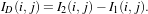(21.1)

In order to make the algorithm robust to noise, one actually uses local means instead of pixel values.

The source code for this example can be found in the file
Examples/ChangeDetection/DiffChDet.cxx.

This example illustrates the class otb::MeanDifferenceImageFilter for detecting changes between pairs of images. This filter computes the mean intensity in the neighborhood of each pixel of the pair of images to be compared and uses the difference of means as a change indicator. This example will use the images shown in figure  21.1. These correspond to the near infrared band of two Spot acquisitions before and during a flood.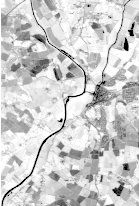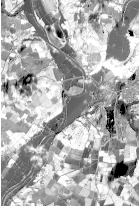Figure 21.1: Images used for the change detection. Left: Before the flood. Right: during the flood.

We start by including the corresponding header file.

#include "otbMeanDifferenceImageFilter.h"

We start by declaring the types for the two input images, the change image and the image to be stored in a file for visualization.

typedef float                                    InternalPixelType;
typedef unsigned char                            OutputPixelType;
typedef otb::Image<InternalPixelType, Dimension> InputImageType1;
typedef otb::Image<InternalPixelType, Dimension> InputImageType2;
typedef otb::Image<InternalPixelType, Dimension> ChangeImageType;
typedef otb::Image<OutputPixelType, Dimension>   OutputImageType;

We can now declare the types for the readers and the writer.

typedef otb::ImageFileWriter<OutputImageType> WriterType;

The change detector will give positive and negative values depending on the sign of the difference. We are usually interested only in the absolute value of the difference. For this purpose, we will use the itk::AbsImageFilter . Also, before saving the image to a file in, for instance, PNG format, we will rescale the results of the change detection in order to use the full range of values of the output pixel type.

typedef itk::AbsImageFilter<ChangeImageType,
ChangeImageType> AbsType;
typedef itk::RescaleIntensityImageFilter<ChangeImageType,

The otb::MeanDifferenceImageFilter is templated over the types of the two input images and the type of the generated change image.

typedef otb::MeanDifferenceImageFilter<
InputImageType1,
InputImageType2,
ChangeImageType>       FilterType;

The different elements of the pipeline can now be instantiated.

WriterType::Pointer   writer = WriterType::New();
FilterType::Pointer   filter = FilterType::New();
AbsType::Pointer      absFilter = AbsType::New();

We set the parameters of the different elements of the pipeline.

writer->SetFileName(outputFilename);
rescaler->SetOutputMinimum(itk::NumericTraits<OutputPixelType>::min());
rescaler->SetOutputMaximum(itk::NumericTraits<OutputPixelType>::max());

The only parameter for this change detector is the radius of the window used for computing the mean of the intensities.

We build the pipeline by plugging all the elements together.

absFilter->SetInput(filter->GetOutput());
rescaler->SetInput(absFilter->GetOutput());
writer->SetInput(rescaler->GetOutput());

Since the processing time of large images can be long, it is interesting to monitor the evolution of the computation. In order to do so, the change detectors can use the command/observer design pattern. This is easily done by attaching an observer to the filter.

typedef otb::CommandProgressUpdate<FilterType> CommandType;

CommandType::Pointer observer = CommandType::New();

Figure 21.2 shows the result of the change detection by difference of local means.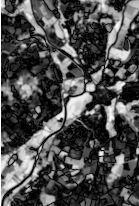Figure 21.2: Result of the mean difference change detector

#### 21.3.2 Ratio Of Means

This detector is similar to the previous one except that it uses a ratio instead of the difference: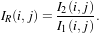(21.2)

The use of the ratio makes this detector robust to multiplicative noise which is a good model for the speckle phenomenon which is present in radar images.

In order to have a bounded and normalized detector the following expression is actually used: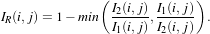(21.3)

The source code for this example can be found in the file
Examples/ChangeDetection/RatioChDet.cxx.

This example illustrates the class otb::MeanRatioImageFilter for detecting changes between pairs of images. This filter computes the mean intensity in the neighborhood of each pixel of the pair of images to be compared and uses the ratio of means as a change indicator. This change indicator is then normalized between 0 and 1 by using the classical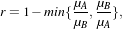(21.4)

where μA and μB are the local means. This example will use the images shown in figure  21.3. These correspond to 2 Radarsat fine mode acquisitions before and after a lava flow resulting from a volcanic eruption.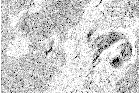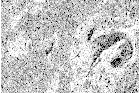Figure 21.3: Images used for the change detection. Left: Before the eruption. Right: after the eruption.

We start by including the corresponding header file.

#include "otbMeanRatioImageFilter.h"

We start by declaring the types for the two input images, the change image and the image to be stored in a file for visualization.

typedef float                                    InternalPixelType;
typedef unsigned char                            OutputPixelType;
typedef otb::Image<InternalPixelType, Dimension> InputImageType1;
typedef otb::Image<InternalPixelType, Dimension> InputImageType2;
typedef otb::Image<InternalPixelType, Dimension> ChangeImageType;
typedef otb::Image<OutputPixelType, Dimension>   OutputImageType;

We can now declare the types for the readers. Since the images can be vey large, we will force the pipeline to use streaming. For this purpose, the file writer will be streamed. This is achieved by using the otb::ImageFileWriter class.

typedef otb::ImageFileWriter<OutputImageType> WriterType;

The change detector will give a normalized result between 0 and 1. In order to store the result in PNG format we will rescale the results of the change detection in order to use all the output pixel type range of values.

typedef itk::ShiftScaleImageFilter<ChangeImageType,

The otb::MeanRatioImageFilter is templated over the types of the two input images and the type of the generated change image.

typedef otb::MeanRatioImageFilter<
InputImageType1,
InputImageType2,
ChangeImageType>       FilterType;

The different elements of the pipeline can now be instantiated.

WriterType::Pointer   writer = WriterType::New();
FilterType::Pointer   filter = FilterType::New();

We set the parameters of the different elements of the pipeline.

writer->SetFileName(outputFilename);
float scale = itk::NumericTraits<OutputPixelType>::max();
rescaler->SetScale(scale);

The only parameter for this change detector is the radius of the window used for computing the mean of the intensities.

We build the pipeline by plugging all the elements together.

rescaler->SetInput(filter->GetOutput());
writer->SetInput(rescaler->GetOutput());

Figure 21.4 shows the result of the change detection by ratio of local means.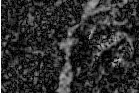Figure 21.4: Result of the ratio of means change detector

### 21.4 Statistical Detectors

#### 21.4.1 Distance between local distributions

This detector is similar to the ratio of means detector (seen in the previous section page 922). Nevertheless, instead of the comparison of means, the comparison is performed to the complete distribution of the two Random Variables (RVs) .

The detector is based on the Kullback-Leibler distance between probability density functions (pdfs). In the neighborhood of each pixel of the pair of images I1 and I2 to be compared, the distance between local pdfs f1 and f2 of RVs X1 and X2 is evaluated by: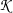(X1,X2) = K(X1|X2)+K(X2|X1) (21.5) with K(Xj|Xi) = ∫ Rlog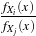fXi(x)dx, i,j = 1,2. (21.6)
In order to reduce the computational time, the local pdfs f1 and f2 are not estimated through histogram computations but rather by a cumulant expansion, namely the Edgeworth expansion, with is based on the cumulants of the RVs: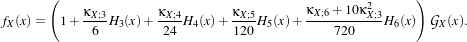(21.7)

In eq. (21.7),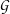X stands for the Gaussian pdf which has the same mean and variance as the RV X. The κX;k coefficients are the cumulants of order k, and Hk(x) are the Chebyshev-Hermite polynomials of order k (see  for deeper explanations).

The source code for this example can be found in the file
Examples/ChangeDetection/KullbackLeiblerDistanceChDet.cxx.

This example illustrates the class otb::KullbackLeiblerDistanceImageFilter for detecting changes between pairs of images. This filter computes the Kullback-Leibler distance between probability density functions (pdfs). In fact, the Kullback-Leibler distance is itself approximated through a cumulant-based expansion, since the pdfs are approximated through an Edgeworth series. The Kullback-Leibler distance is evaluated by: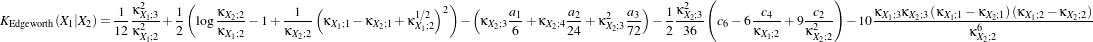(21.8)
where
 a1 = c3 -3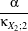a2 = c4 -6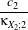+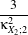a3 = c6 -15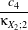+45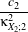-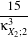c2 = α2 +β2c3 = α3 +3αβ2c4 = α4 +6α2β2 +3β4c 6 = α6 +15α4β2 +45α2β4 +15β6α =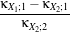β =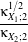.
κXi;1, κXi;2, κXi;3 and κXi;4 are the cumulants up to order 4 of the random variable Xi (i = 1,2). This example will use the images shown in figure 21.3. These correspond to 2 Radarsat fine mode acquisitions before and after a lava flow resulting from a volcanic eruption.

The program itself is very similar to the ratio of means detector, implemented in otb::MeanRatioImageFilter , in section 21.3.2. Nevertheless the corresponding header file has to be used instead.

#include "otbKullbackLeiblerDistanceImageFilter.h"

The otb::KullbackLeiblerDistanceImageFilter is templated over the types of the two input images and the type of the generated change image, in a similar way as the otb::MeanRatioImageFilter . It is the only line to be changed from the ratio of means change detection example to perform a change detection through a distance between distributions...

typedef otb::KullbackLeiblerDistanceImageFilter<ImageType,
ImageType,
ImageType> FilterType;

The different elements of the pipeline can now be instantiated. Follow the ratio of means change detector example.

The only parameter for this change detector is the radius of the window used for computing the cumulants.

FilterType::Pointer filter = FilterType::New();

The pipeline is built by plugging all the elements together.

Figure 21.5 shows the result of the change detection by computing the Kullback-Leibler distance between local pdf through an Edgeworth approximation.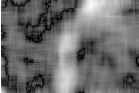Figure 21.5: Result of the Kullback-Leibler change detector

#### 21.4.2 Local Correlation

The correlation coefficient measures the likelihood of a linear relationship between two random variables: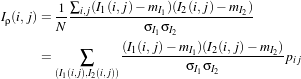(21.9)

where I1(i,j) and I2(i,j) are the pixel values of the 2 images and pij is the joint probability density. This is like using a linear model: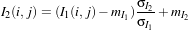(21.10)

for which we evaluate the likelihood with pij.

With respect to the difference detector, this one will be robust to illumination changes.

The source code for this example can be found in the file
Examples/ChangeDetection/CorrelChDet.cxx.

This example illustrates the class otb::CorrelationChangeDetector for detecting changes between pairs of images. This filter computes the correlation coefficient in the neighborhood of each pixel of the pair of images to be compared. This example will use the images shown in figure  21.6. These correspond to two ERS acquisitions before and during a flood.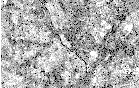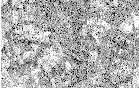Figure 21.6: Images used for the change detection. Left: Before the flood. Right: during the flood.

We start by including the corresponding header file.

#include "otbCorrelationChangeDetector.h"

We start by declaring the types for the two input images, the change image and the image to be stored in a file for visualization.

typedef float                                    InternalPixelType;
typedef unsigned char                            OutputPixelType;
typedef otb::Image<InternalPixelType, Dimension> InputImageType1;
typedef otb::Image<InternalPixelType, Dimension> InputImageType2;
typedef otb::Image<InternalPixelType, Dimension> ChangeImageType;
typedef otb::Image<OutputPixelType, Dimension>   OutputImageType;

We can now declare the types for the readers. Since the images can be vey large, we will force the pipeline to use streaming. For this purpose, the file writer will be streamed. This is achieved by using the otb::ImageFileWriter class.

typedef otb::ImageFileWriter<OutputImageType> WriterType;

The change detector will give a response which is normalized between 0 and 1. Before saving the image to a file in, for instance, PNG format, we will rescale the results of the change detection in order to use all the output pixel type range of values.

typedef itk::ShiftScaleImageFilter<ChangeImageType,

The otb::CorrelationChangeDetector is templated over the types of the two input images and the type of the generated change image.

typedef otb::CorrelationChangeDetector<
InputImageType1,
InputImageType2,
ChangeImageType>       FilterType;

The different elements of the pipeline can now be instantiated.

WriterType::Pointer   writer = WriterType::New();
FilterType::Pointer   filter = FilterType::New();

We set the parameters of the different elements of the pipeline.

writer->SetFileName(outputFilename);

float scale = itk::NumericTraits<OutputPixelType>::max();
rescaler->SetScale(scale);

The only parameter for this change detector is the radius of the window used for computing the correlation coefficient.

We build the pipeline by plugging all the elements together.

rescaler->SetInput(filter->GetOutput());
writer->SetInput(rescaler->GetOutput());

Since the processing time of large images can be long, it is interesting to monitor the evolution of the computation. In order to do so, the change detectors can use the command/observer design pattern. This is easily done by attaching an observer to the filter.

typedef otb::CommandProgressUpdate<FilterType> CommandType;

CommandType::Pointer observer = CommandType::New();

Figure 21.7 shows the result of the change detection by local correlation.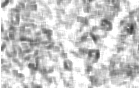Figure 21.7: Result of the correlation change detector

### 21.5 Multi-Scale Detectors

#### 21.5.1 Kullback-Leibler Distance between distributions

This technique is an extension of the distance between distributions change detector presented in section 21.4.1. Since this kind of detector is based on cumulants estimations through a sliding window, the idea is just to upgrade the estimation of the cumulants by considering new samples as soon as the sliding window is increasing in size.

Let’s consider the following problem: how to update the moments when a N +1th observation xN+1 is added to a set of observations {x1,x2,,xN} already considered. The evolution of the central moments may be characterized by:

 μ1,[N] =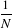s1,[N] (21.11) μr,[N] =∑ℓ=0r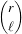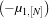r-ℓs ℓ,[N],
where the notation sr,[N] = i=1Nxir has been used. Then, Edgeworth series is updated also by transforming moments to cumulants by using: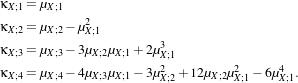(21.12)

It yields a set of images that represent the change measure according to an increasing size of the analysis window.

The source code for this example can be found in the file
Examples/ChangeDetection/KullbackLeiblerProfileChDet.cxx.

This example illustrates the class otb::KullbackLeiblerProfileImageFilter for detecting changes between pairs of images, according to a range of window size. This example is very similar, in its principle, to all of the change detection examples, especially the distance between distributions one (section 21.4.1) which uses a fixed window size.

The main differences are:

1. a set of window range instead of a fixed size of window;
2. an output of type otb::VectorImage .

Then, the program begins with the otb::VectorImage and the otb::KullbackLeiblerProfileImageFilter header files in addition to those already details in the otb::MeanRatioImageFilter example.

#include "otbKullbackLeiblerProfileImageFilter.h"

The otb::KullbackLeiblerProfileImageFilter is templated over the types of the two input images and the type of the generated change image (which is now of multi-components), in a similar way as the otb::KullbackLeiblerDistanceImageFilter .

typedef otb::Image<PixelType, Dimension>       ImageType;
typedef otb::VectorImage<PixelType, Dimension> VectorImageType;
typedef otb::KullbackLeiblerProfileImageFilter<ImageType,
ImageType,
VectorImageType> FilterType;

The different elements of the pipeline can now be instantiated in the same way as the ratio of means change detector example.

Two parameters are now required to give the minimum and the maximum size of the analysis window. The program will begin by performing change detection through the smaller window size and then applying moments update of eq. (21.11) by incrementing the radius of the analysis window (i.e. add a ring of width 1 pixel around the current neightborhood shape). The process is applied until the larger window size is reached.

FilterType::Pointer filter = FilterType::New();
filter->SetRadius((winSizeMin - 1) / 2, (winSizeMax - 1) / 2);

Figure 21.8 shows the result of the change detection by computing the Kullback-Leibler distance between local pdf through an Edgeworth approximation.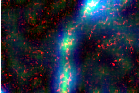Figure 21.8: Result of the Kullback-Leibler profile change detector, colored composition including the first, 12th and 24th channel of the generated output.

### 21.6 Multi-components detectors

#### 21.6.1 Multivariate Alteration Detector

The source code for this example can be found in the file
Examples/ChangeDetection/MultivariateAlterationDetector.cxx.

This example illustrates the class otb::MultivariateAlterationChangeDetectorImageFilter , which implements the Multivariate Alteration Change Detector algorithm . This algorihtm allows performing change detection from a pair multi-band images, including images with different number of bands or modalities. Its output is a a multi-band image of change maps, each one being unccorrelated with the remaining. The number of bands of the output image is the minimum number of bands between the two input images.

The algorithm works as follows. It tries to find two linear combinations of bands (one for each input images) which maximize correlation, and subtract these two linear combinitation, leading to the first change map. Then, it looks for a second set of linear combinations which are orthogonal to the first ones, a which maximize correlation, and use it as the second change map. This process is iterated until no more orthogonal linear combinations can be found.

This algorithms has numerous advantages, such as radiometry scaling and shifting invariance and absence of parameters, but it can not be used on a pair of single band images (in this case the output is simply the difference between the two images).

We start by including the corresponding header file.

#include "otbMultivariateAlterationDetectorImageFilter.h"

We then define the types for the input images and for the change image.

typedef unsigned short                               InputPixelType;
typedef float                                        OutputPixelType;
typedef otb::VectorImage<InputPixelType, Dimension>  InputImageType;
typedef otb::VectorImage<OutputPixelType, Dimension> OutputImageType;

We can now declare the types for the reader. Since the images can be vey large, we will force the pipeline to use streaming. For this purpose, the file writer will be streamed. This is achieved by using the otb::ImageFileWriter class.

typedef otb::ImageFileWriter<OutputImageType> WriterType;

typedef otb::MultivariateAlterationDetectorImageFilter<

The different elements of the pipeline can now be instantiated.

WriterType::Pointer    writer = WriterType::New();

We set the parameters of the different elements of the pipeline.

writer->SetFileName(outputFilename);

We build the pipeline by plugging all the elements together.